•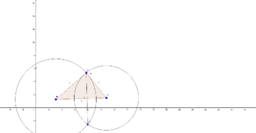Construct the Altitude of the Triangle

Activity

•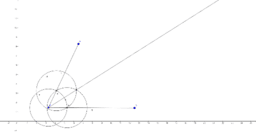Geometric Constructions Using GeoGebra

Book

•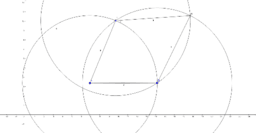Construction of a Kite

Activity

•Construction of a Rhombus

Activity

•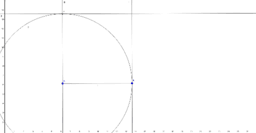Construction of a Square

Activity

•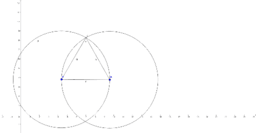Construction of an Equilateral Triangle

Activity

•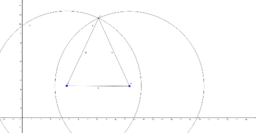Construction of an Isosceles Triangle

Activity

•Construction of an Angle Bisector

Activity

•Construction of an Angle Bisector

Activity

•Construction of an Angle Bisector

Activity

•Construction of an Angle Bisector

Activity

•Construction of an Angle Bisector

Activity

•